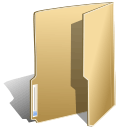## Conversion (24)

Wednesday, 24 October 2018 08:56

### How to save Excel chart sheet to SVG in C#

Written by support iceblue

A ChartSheet represents a chart sheet. It is a worksheet that contains only a chart. This article will demonstrate how to convert a chart sheet to SVG stream by using Spire.XLS.

Firstly, view the sample Excel worksheets with two chart sheets.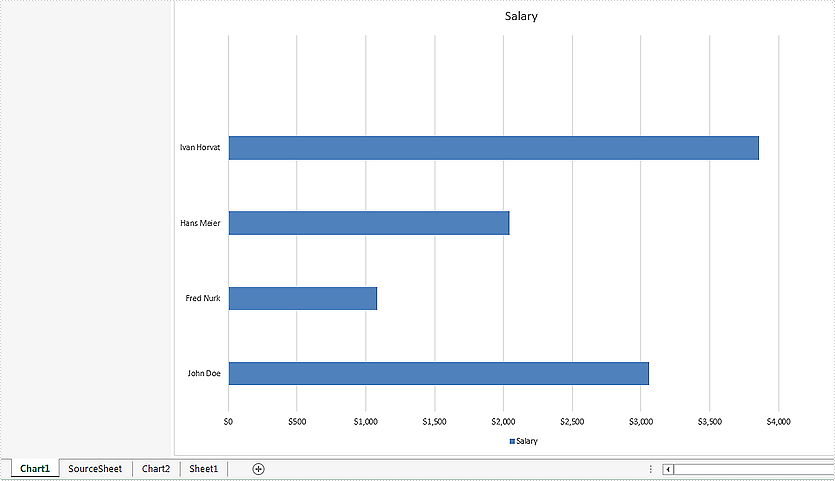Convert all the chart sheets to SVG stream:

```//load the document from file
Workbook workbook = new Workbook();

//call ToSVGStream(Stream stream) method to save each chart sheet to SVG stream
for (int i = 0; i < workbook.Chartsheets.Count; i++)
{
FileStream fs = new FileStream(string.Format("chartsheet-{0}.svg", i), FileMode.Create);
workbook.Chartsheets[i].ToSVGStream(fs);
fs.Flush();
fs.Close();
}
```

Effective screenshot of the two chart sheets to SVG.```//load the document from file
Workbook workbook = new Workbook();

//get the second chartsheet by name
ChartSheet cs = workbook.GetChartSheetByName("Chart2");

//save to SVG stream
FileStream fs = new FileStream(string.Format("chart2.svg"), FileMode.Create);
cs.ToSVGStream(fs);
fs.Flush();
fs.Close();
```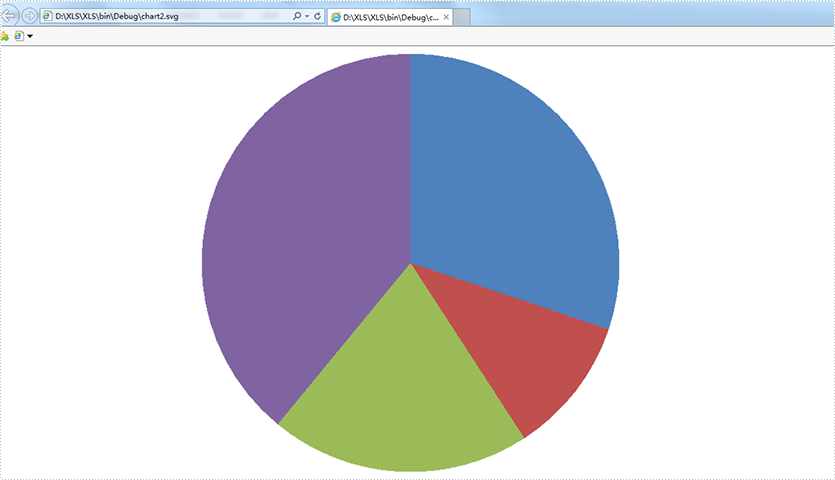Tuesday, 18 September 2018 07:58

### Convert a Chart Sheet to Image in C#

Written by support iceblue

A chart sheet is a separate worksheet that only contains chart, at some times we may need to convert chart sheet to image. This article is going to elaborates how we can convert a chart sheet to image using Spire.XLS.

Screenshot of the Chart sheet: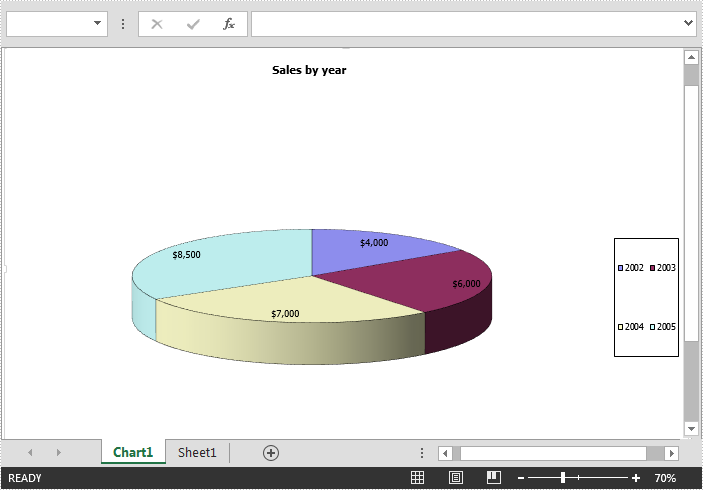Detail steps:

Step 1: Instantiate a Workbook object and load the excel file.

```Workbook workbook = new Workbook();
```

Step 2: Get the first chart sheet.

```ChartSheet chartSheet = workbook.Chartsheets;
```

Step 3: Save the chart sheet to image and save the image to disk.

```Image image = workbook.SaveChartAsImage(chartSheet);
image.Save("ChartSheet.png", ImageFormat.Png);
```

Output image: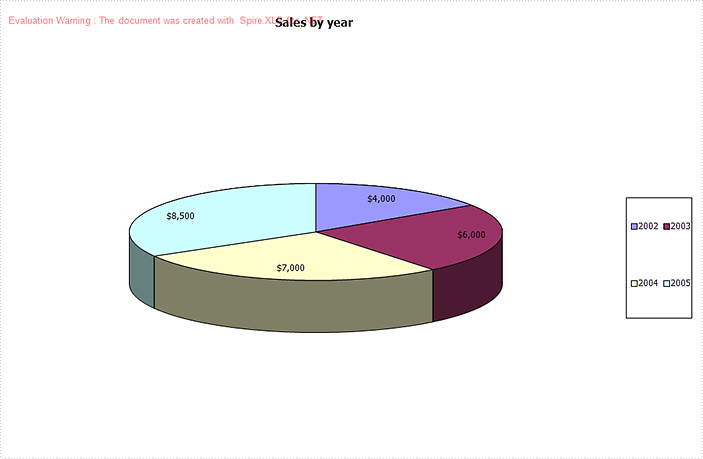Full code:

```//Instantiate a Workbook object
Workbook workbook = new Workbook();

//Get the first chart sheet
ChartSheet chartSheet = workbook.Chartsheets;

//Save the chart sheet as image
Image image = workbook.SaveChartAsImage(chartSheet);
//Save image
image.Save("ChartSheet.png", ImageFormat.Png);
```
Wednesday, 28 February 2018 08:29

### Convert Excel Sheet to a High-Resolution Image in C#, VB.NET

Written by support iceblue

Sometimes, you may want to convert Excel sheet to a high-resolution image, especially when the Excel report contains graphs or pictures. This article will show you how to set image resolution when saving Excel sheet to JPG using Spire.XLS.

Step 1: Create a custom function that you can use to reset the image resolution.

```private static Bitmap ResetResolution(Metafile mf, float resolution)
{
int width = (int)(mf.Width * resolution / mf.HorizontalResolution);
int height = (int)(mf.Height * resolution / mf.VerticalResolution);
Bitmap bmp = new Bitmap(width, height);
bmp.SetResolution(resolution, resolution);
Graphics g = Graphics.FromImage(bmp);
g.DrawImage(mf, 0, 0);
g.Dispose();
return bmp;
}
```

Step 2: Create a workbook instance and load the sample Excel file.

```Workbook workbook = new Workbook();
```

Step 3: Get the worksheet you want to convert.

```Worksheet worksheet = workbook.Worksheets;
```

Step 4: Convert the worksheet to EMF stream.

```MemoryStream ms = new MemoryStream();
worksheet.ToEMFStream(ms, 1, 1, worksheet.LastRow, worksheet.LastColumn);
```

Step 5: Create an image from the EMF stream, and call ResetResolution to reset the resolution for the image.

```Image image = Image.FromStream(ms);
Bitmap images = ResetResolution(image as Metafile, 300);
```

Step 6: Save the image in JPG file format.

```images.Save("Result.jpg", ImageFormat.Jpeg);
```

Output: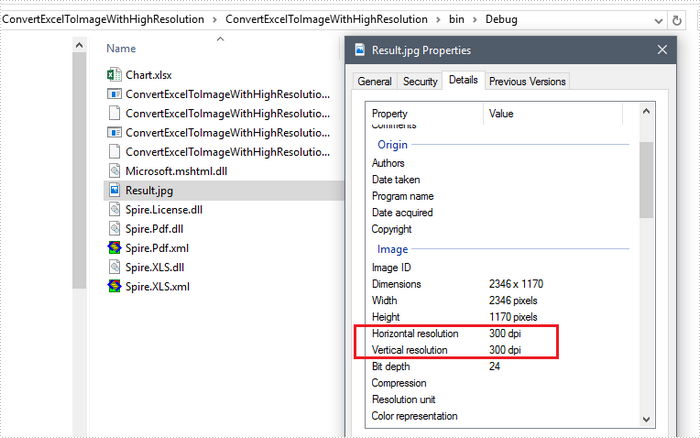Full Code:

[C#]
```static void Main(string[] args)
{
Workbook workbook = new Workbook();
Worksheet worksheet = workbook.Worksheets;

using (MemoryStream ms = new MemoryStream())
{

worksheet.ToEMFStream(ms, 1, 1, worksheet.LastRow, worksheet.LastColumn);
Image image = Image.FromStream(ms);
Bitmap images = ResetResolution(image as Metafile, 300);
images.Save("Result.jpg", ImageFormat.Jpeg);
}
}
private static Bitmap ResetResolution(Metafile mf, float resolution)
{
int width = (int)(mf.Width * resolution / mf.HorizontalResolution);
int height = (int)(mf.Height * resolution / mf.VerticalResolution);
Bitmap bmp = new Bitmap(width, height);
bmp.SetResolution(resolution, resolution);
Graphics g = Graphics.FromImage(bmp);
g.DrawImage(mf, 0, 0);
g.Dispose();
return bmp;
}
```
[VB.NET]
```Shared Sub Main(ByVal args() As String)
Dim workbook As Workbook = New Workbook()
Dim worksheet As Worksheet = workbook.Worksheets(0)

Imports(MemoryStream ms = New MemoryStream())
{

worksheet.ToEMFStream(ms, 1, 1, worksheet.LastRow, worksheet.LastColumn)
Dim image As Image = Image.FromStream(ms)
Dim images As Bitmap = ResetResolution(image as Metafile, 300)
images.Save("Result.jpg", ImageFormat.Jpeg)
}
End Sub
Private Shared Function ResetResolution(ByVal mf As Metafile, ByVal resolution As single) As Bitmap
Dim width As Integer = CType((mf.Width * resolution / mf.HorizontalResolution), Integer)
Dim height As Integer = CType((mf.Height * resolution / mf.VerticalResolution), Integer)
Dim bmp As Bitmap = New Bitmap(width, height)
bmp.SetResolution(resolution, resolution)
Dim g As Graphics = Graphics.FromImage(bmp)
g.DrawImage(mf, 0, 0)
g.Dispose()
Return bmp
End Function
```
Tuesday, 10 October 2017 08:46

### How to Convert CSV to PDF in C#, VB.NET

Written by support iceblue

Spire.XLS supports to load a CSV file and save as an Excel or PDF file. This tutorial shows how to convert CSV to PDF using Spire.XLS with C# and VB.NET.

The sample CSV file looks as follows.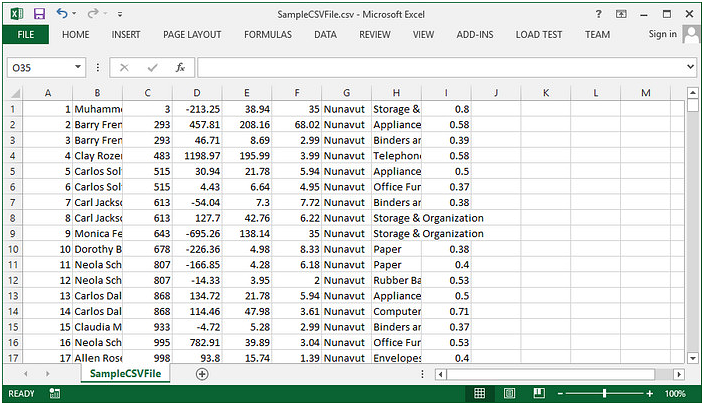Step 1: Create a Workbook instance and load a sample CSV file.

```Workbook wb = new Workbook();
```

Step 2: To render content of a worksheet into a single PDF page, set the SheetFitToPage property as true.

```wb.ConverterSetting.SheetFitToPage = true;
```

Step 3: Autofit a column if the characters in the column exceed column width.

```Worksheet sheet = wb.Worksheets;
for (int i = 1; i < sheet.Columns.Length; i++)
{
sheet.AutoFitColumn(i);
}
```

Step 4: Save the specified worksheet to PDF.

```sheet.SaveToPdf("toPDF.pdf");
```

Output:Full Code:

[C#]
```Workbook wb = new Workbook();
wb.ConverterSetting.SheetFitToPage = true;
Worksheet sheet = wb.Worksheets;
for (int i = 1; i < sheet.Columns.Length; i++)
{
sheet.AutoFitColumn(i);
}
sheet.SaveToPdf("toPDF.pdf");
```
[VB.NET]
```Dim wb As New Workbook()
wb.ConverterSetting.SheetFitToPage = True
Dim sheet As Worksheet = wb.Worksheets(0)
For i As Integer = 1 To sheet.Columns.Length - 1
sheet.AutoFitColumn(i)
Next
sheet.SaveToPdf("toPDF.pdf")
```
Monday, 24 October 2016 08:12

### How to Convert Excel Worksheet to SVG (Scalable Vector Graphics) in C#, VB.NET

Written by support iceblue

In this article, we will introduce the way of converting Excel worksheet to SVG(Scalable Vector Graphics) file format in C# and VB.NET with the help of Spire.XLS, which is a 100% standalone Excel .NET API that allows developers to create and manipulate Excel spreadsheets without requiring Microsoft Excel or Microsoft Office to be installed on system.

Please see the detail steps in the following contents.

Step 1: Instantiate a Workbook object and load the Excel workbook.

```Workbook workbook = new Workbook();
```

Step 2: Create a file stream, call ToSVGStream(Stream stream, int firstRow, int firstColumn, int lastRow, int lastColumn) method to save each worksheet of the workbook to SVG stream and then save to the file stream. Finally, close the file stream.

```for (int i = 0; i < workbook.Worksheets.Count; i++)
{
FileStream fs = new FileStream(string.Format("E:\\Program Files\\sheet-{0}.svg", i), FileMode.Create);
workbook.Worksheets[i].ToSVGStream(fs, 0, 0, 0, 0);
fs.Flush();
fs.Close();
}
```

Effective screenshot:

For better demonstration, we used a sample Excel workbook which contains two worksheets, below are the comparisons between the source worksheets and the result SVG files after converting.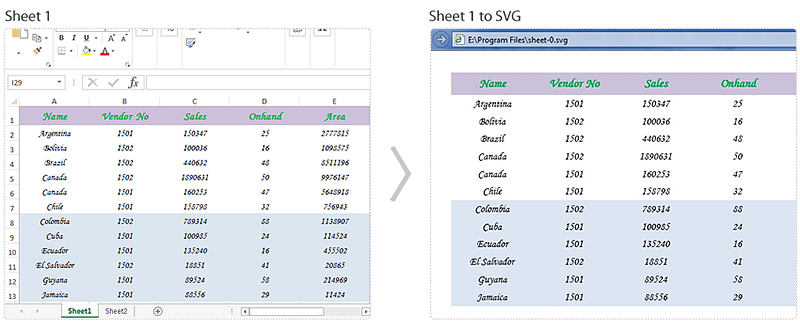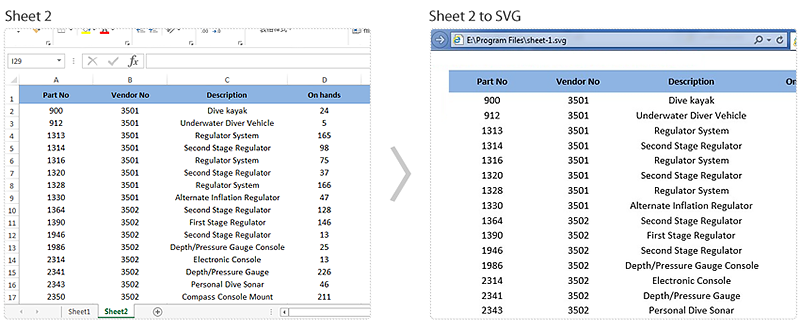Full code:

[C#]
```using System.IO;
using Spire.Xls;

namespace Convert_Excel_to_SVG
{
class Program
{
static void Main(string[] args)
{
Workbook workbook = new Workbook();
for (int i = 0; i < workbook.Worksheets.Count; i++)
{
FileStream fs = new FileStream(string.Format("E:\\Program Files\\sheet-{0}.svg", i), FileMode.Create);
workbook.Worksheets[i].ToSVGStream(fs, 0, 0, 0, 0);
fs.Flush();
fs.Close();
}
}
}
}
```
[VB.NET]
```Imports System.IO
Imports Spire.Xls

Namespace Convert_Excel_to_SVG
Class Program
Private Shared Sub Main(args As String())
Dim workbook As New Workbook()
For i As Integer = 0 To workbook.Worksheets.Count - 1
Dim fs As New FileStream(String.Format("sheet-{0}.svg", i), FileMode.Create)
workbook.Worksheets(i).ToSVGStream(fs, 0, 0, 0, 0)
fs.Flush()
fs.Close()
Next
End Sub
End Class
End Namespace
```
Wednesday, 10 August 2016 03:17

### How to Convert Excel to OpenDocument Spreadsheet (.ods) format in C#, VB.NET

Written by support iceblue

The OpenDocument Spreadsheet format (commonly referred to as ODS format) is an XML-based file format for editable spreadsheet documents defined by OASIS. This free ISO-standardized format is now widely supported by a variety of free software applications, such as OpenOffice.org and the LibreOffice suite.

#### Using C#/VB.NET to convert Excel to .ods format via Spire.XLS

Spire.XLS, a powerful .NET excel component, enables developers to convert excel documents to ods format easily. This part will introduce you the solution of converting excel to .ods format in c# and vb.net.

Now, please view the following screenshot of the original excel document in .xlsx format: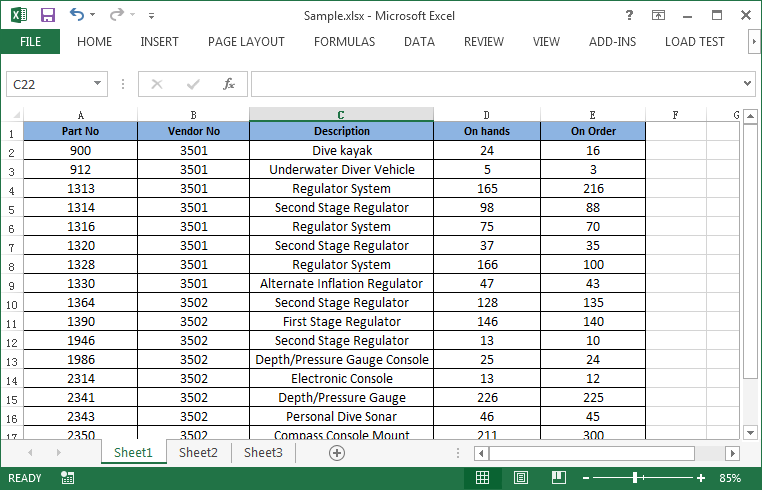Then refer to the following steps:

Step 1: Initialize a new instance of Workbook class and load the excel document from file.

```Workbook workbook = new Workbook();
```

Step 2: Invoke Workbook.SaveToFile(string fileName, FileFormat fileFormat) method to save the excel document to ODS format.

```workbook.SaveToFile("Result.ods",FileFormat.ODS);
```

Run the project, we will get the following result file in .ods format: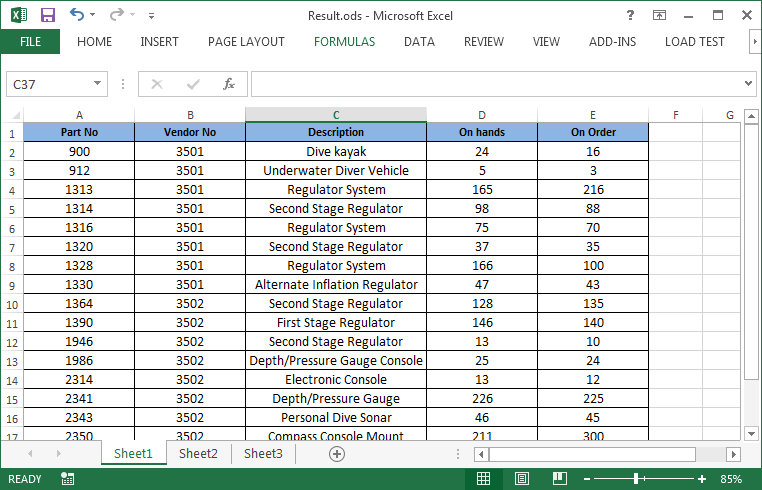Full codes:

[C#]
```using Spire.Xls;

namespace Convert_Excel_to_ODS
{
class Program
{
static void Main(string[] args)
{
Workbook workbook = new Workbook();
workbook.SaveToFile("Result.ods",FileFormat.ODS);
}
}
}
```
[VB.NET]
```Imports Spire.Xls

Namespace Convert_Excel_to_ODS
Class Program
Private Shared Sub Main(args As String())
Dim workbook As New Workbook()
workbook.SaveToFile("Result.ods", FileFormat.ODS)
End Sub
End Class
End Namespace=
```
Friday, 04 March 2016 07:26

### Convert worksheet to PDF in C#, VB.NET

Written by support iceblue

We have discussed before about converting workbook to PDF. However, in this section, we will show you a neat solution to convert the specific worksheet to PDF with C# and VB.NET in workbook. Apply Spire.Xls for .NET in your application and you can turn worksheet into PDF easily without changing the layout of worksheet.

In the following sections, we will demonstrate how to convert worksheet to PDF.

Step 1: Initialize a new instance of Workbook class and load the sample Excel file.

```Workbook workbook = new Workbook();
```

Step 2: Get its first worksheet.

```Worksheet sheet = workbook.Worksheets;
```

Step 3: Convert the selected worksheet to PDF and save to file.

```sheet.SaveToPdf("toPDF.pdf");
```

Step 4: Launch the file.

```System.Diagnostics.Process.Start("toPDF.pdf");
```

Effective screenshot: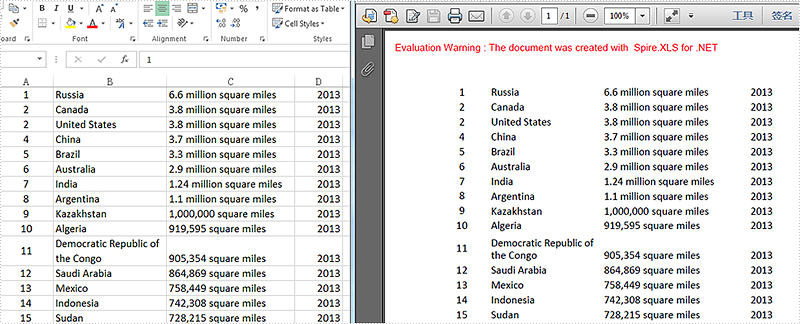Full codes:

[C#]
```using Spire.Xls;

namespace Excel_Worksheet_to_PDF
{
class Program
{
static void Main(string[] args)
{
Workbook workbook = new Workbook();

Worksheet sheet = workbook.Worksheets;

sheet.SaveToPdf("toPDF.pdf");
System.Diagnostics.Process.Start("toPDF.pdf");
}
}
}
```
[VB.NET]
```Imports Spire.Xls

Namespace Excel_Worksheet_to_PDF
Class Program
Private Shared Sub Main(args As String())
Dim workbook As New Workbook()

Dim sheet As Worksheet = workbook.Worksheets(0)

sheet.SaveToPdf("toPDF.pdf")
System.Diagnostics.Process.Start("toPDF.pdf")
End Sub
End Class
End Namespace
```
Friday, 10 July 2015 05:37

### Show formula and its result separately when converting excel to datatable in C#

Written by support iceblue

This article shows how to display formula and its result separately when converting excel to database via Spire.XLS. This demo uses an Excel file with formula in it and show the conversion result in a Windows Forms Application project.

Screenshot of the test excel file: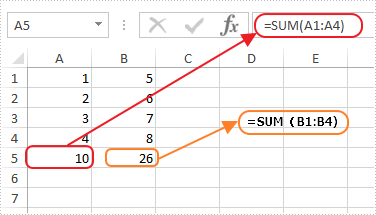Here are the detailed steps:

Steps 1: Create a Windows Forms Application in Visual Studio.

Steps 2: Drag a DataGridView and two Buttons from Toolbox to the Form and change names of the buttons as Formula and Result to distinguish.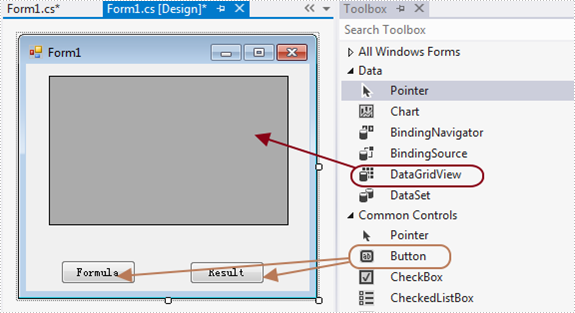Steps 3: Double click Button formula and add the following code.

3.1 Load test file and get the first sheet.

```Workbook workbook = new Workbook();
Worksheet sheet = workbook.Worksheets;
```

3.2 Invoke method ExportDataTable of the sheet and output data range. Parameters of ExportDataTable are range to export, indicates if export column name and indicates whether compute formula value, then it will return exported datatable.

Description of ExportDataTable:

```public DataTable ExportDataTable(CellRange range, bool exportColumnNames, bool computedFormulaValue);
```

Code:

```DataTable dt = sheet.ExportDataTable(sheet.AllocatedRange, false, false);
```

3.3 Show in DataGridView

`this.dataGridView1.DataSource = dt; `

Steps 4: Do ditto to Button Result. Only alter parameter computedFormulaValue as true.

```Workbook workbook = new Workbook();
Worksheet sheet = workbook.Worksheets;
DataTable dt = sheet.ExportDataTable(sheet.AllocatedRange, false, true);
this.dataGridView1.DataSource = dt; ```

Steps 5: Start the project and check the result.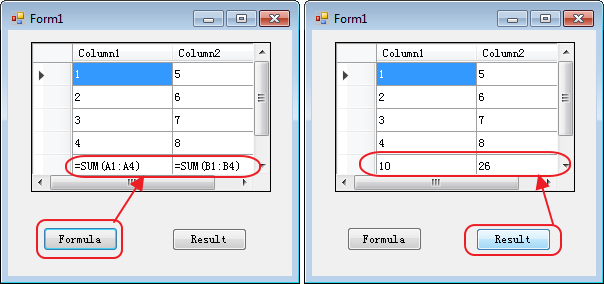Button code here:

```//Formula
private void button1_Click(object sender, EventArgs e)
{
Workbook workbook = new Workbook();
Worksheet sheet = workbook.Worksheets;
DataTable dt = sheet.ExportDataTable(sheet.AllocatedRange, false, false);
this.dataGridView1.DataSource = dt;

}
//Result
private void button2_Click(object sender, EventArgs e)
{
Workbook workbook = new Workbook();
Worksheet sheet = workbook.Worksheets;
DataTable dt = sheet.ExportDataTable(sheet.AllocatedRange, false, true);
this.dataGridView1.DataSource = dt;
}
```
Tuesday, 21 April 2015 08:05

### How to convert Excel worksheet to EMF image in C#

Written by support iceblue

Spire.XLS has powerful functions to export Excel worksheets into different image file formats. In the previous articles, we have already shown you how to convert Excel worksheets into BMP, PNG, GIF, JPG, JPEG, TIFF. Now Spire.XLS newly starts to support exporting Excel worksheet into EMF image. With the help of Spire.XLS, you only need three lines of codes to finish the conversion function.

Make sure Spire.XLS (Version 7.6.43 or above) has been installed correctly and then add Spire.xls.dll as reference in the downloaded Bin folder though the below path: "..\Spire.Xls\Bin\NET4.0\ Spire. Xls.dll". Here comes to the details of how to convert excel worksheet to EMF image.

Step 1: Create an excel document and load the document from file.

```Workbook workbook = new Workbook();
```

Step 2: Get the first worksheet in excel workbook.

```Worksheet sheet = workbook.Worksheets;
```

Step 3: Save excel worksheet into EMF image.

```sheet.SaveToEMFImage("result.emf", 1, 1, 19, 6, system.Drawing.Imaging.EmfType.EmfPlusDual);
```

Effective screenshot: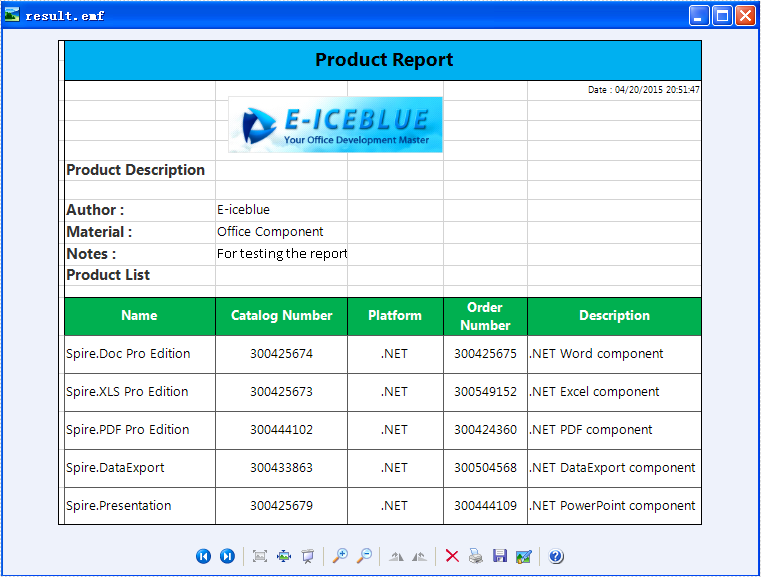Full codes:

```using Spire.Xls;

namespace XLStoEMF
{
class Program
{
static void Main(string[] args)
{
Workbook workbook = new Workbook();

Worksheet sheet = workbook.Worksheets;
sheet.SaveToEMFImage("result.emf", 1, 1, 19,6, System.Drawing.Imaging.EmfType.EmfPlusDual);

}
}
}
```
Friday, 10 April 2015 01:56

### How to Convert Selected Range of Cells to PDF in C#, VB.NET

Written by support iceblue

Using Spire.XLS, programmers are able to save the whole worksheet as PDF by calling the method SaveToPdf(). However, you may only want to save or export a part of worksheet as PDF. Since Spire.XLS doesn't provide a method to directly convert a range of cells to PDF, we can copy the selected ranges to a new worksheet and then save it as PDF file. This method seems complex, but it is still efficient with Spire.XLS.

Look at the test file below, we only want the cells from A1 to H11 converted as PDF. We will firstly create a new blank worksheet, copy the selected range to the new sheet using CellRange.Copy() method, then convert the new sheet as PDF.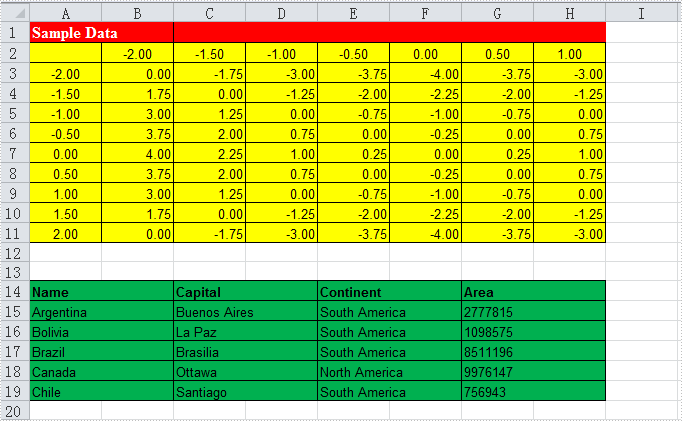Code Snippet:

Step 1: Create a new workbook and load the test file.

```Workbook workbook = new Workbook();
```

Step 2: Add a new worksheet to workbook.

```workbook.Worksheets.Add("newsheet");
```

Step 3: Copy the selected range from where it stores to the new worksheet.

```workbook.Worksheets.Range["A1:H11"].Copy(workbook.Worksheets.Range["A1:H11"]);
```

Step 4: Convert the new worksheet to PDF.

```workbook.Worksheets.SaveToPdf("result.pdf", Spire.Xls.FileFormat.PDF);
```

Result: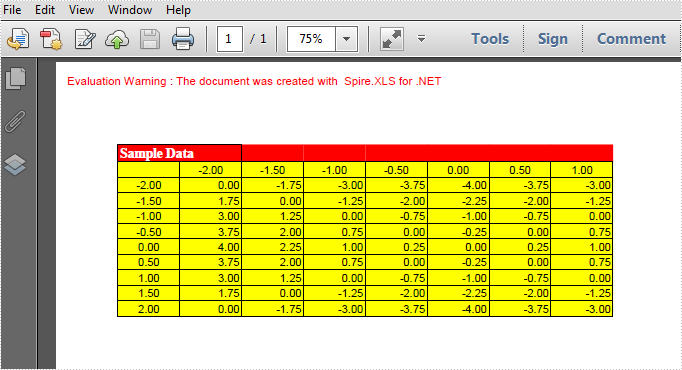Full Code:

[C#]
```Workbook workbook = new Workbook();
// add a new sheet to workbook
//Copy your area to new sheet.
workbook.Worksheets.Range["A1:H11"].Copy(workbook.Worksheets.Range["A1:H11"]);
//convert new sheet to pdf
workbook.Worksheets.SaveToPdf("result.pdf", Spire.Xls.FileFormat.PDF);
```
[VB.NET]
```Dim workbook As New Workbook()